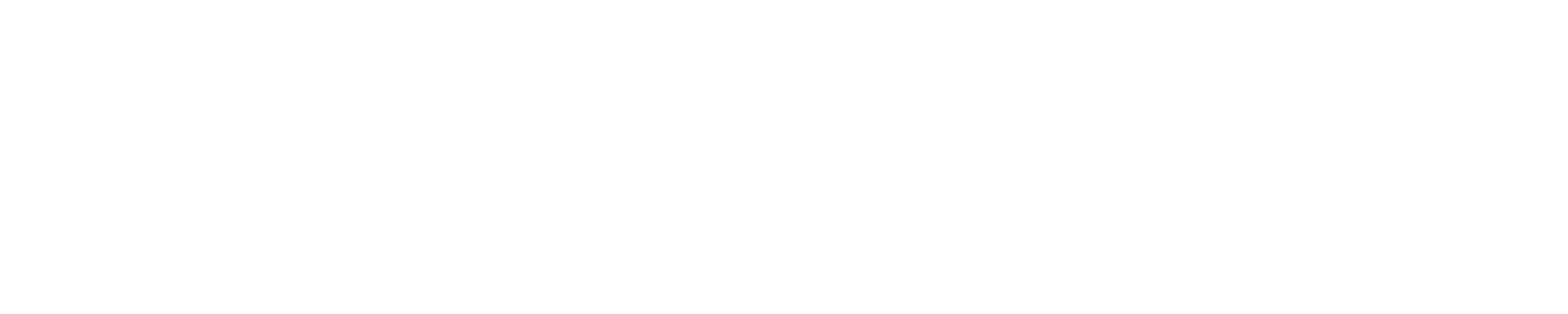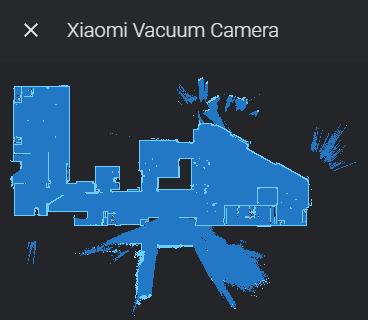# Roborock Live Map Generator

Hi,

I am currently in the process of integrating my rooted Roborock S5 into Home Assistant. Because I really dislike the solutions using a server within Docker Container to display a live map in Home Assistant, I wrote a python script, which takes the map data provided by the Valetudos /api/map/latest endpoint and generates the map out of it. It lacks some polish but now does what it should.

Since Home Assistant is also Python based I was thinking if it might be worth integrating the script into the vacuum/xiaomi Home Assistant component as a sensor/camera, so that external solutions would become unnecessary? Is this viable? I had a look into the git repo of Home Assistant and I was somewhat overwhelmed, so I would need help to do that. In the meantime I leave the script here, so tell me what you think.

``````import io
import json
import requests
from PIL import Image, ImageDraw
import cairosvg

HOST = "192.168.1.43"  # IP of the vacuum
SCALE_FACTOR = 4 # Map scaling
CROP = False # Crop map size to content
DRAW_PATH = True
DRAW_NO_GO_AREAS = True

COLOR_FLOOR = (0, 118, 255)
COLOR_OBSTACLE_WEAK = (0, 0, 0)
COLOR_OBSTACLE_STRONG = (82, 174, 255)
COLOR_PATH = (255, 255, 255)
COLOR_NOGO_BORDER = (255, 0, 0)
COLOR_NOGO_AREA = (255, 0, 0, 128)
COLOR_BACKGROUND = (255, 0, 0, 0)

def transfrom_coords(coord, offset_from):
coord /= 50
coord -= offset_from
coord *= SCALE_FACTOR
return round(coord)

def main():

r = requests.get("http://%s/api/map/latest" % HOST)

# map data
dimensions = json_file["image"]["dimensions"]
floor = json_file["image"]["pixels"]["floor"]
obstacle_weak = json_file["image"]["pixels"]["obstacle_weak"]
obstacle_strong = json_file["image"]["pixels"]["obstacle_strong"]
no_go_areas = json_file["no_go_areas"]

# robot data
robot = json_file["robot"]
charger = json_file["charger"]
path = json_file["path"]["points"]
position = json_file["image"]["position"]
current_angle = json_file["path"]["current_angle"]

vacuum_byte_obj = io.BytesIO()
charger_byte_obj = io.BytesIO()
cairosvg.svg2png(url="robot-vacuum.svg", write_to=vacuum_byte_obj, scale=10)
vacuum = Image.open(vacuum_byte_obj)
vacuum = vacuum.rotate(360-current_angle) # correct for inverse rotation
cairosvg.svg2png(url="flash.svg", write_to=charger_byte_obj, scale=(0.4 * SCALE_FACTOR))
d_station = Image.open(charger_byte_obj)

# draw map
map = Image.new("RGBA", (dimensions["width"], dimensions["height"]), color=COLOR_BACKGROUND)
draw = ImageDraw.Draw(map)

max_x = 0
max_y = 0
min_x = dimensions["width"]
min_y = dimensions["height"]

for coordinate in floor:
# store min/max coordinates for cropping
if CROP:
max_x = coordinate if max_x < coordinate else max_x
max_y = coordinate if max_y < coordinate else max_y
min_x = coordinate if min_x > coordinate else min_x
min_y = coordinate if min_y > coordinate else min_y

draw.point((coordinate,coordinate), fill=COLOR_FLOOR)
for coordinate in obstacle_weak:
draw.point((coordinate,coordinate), fill=COLOR_OBSTACLE_WEAK)
for coordinate in obstacle_strong:
draw.point((coordinate,coordinate), fill=COLOR_OBSTACLE_STRONG)

map = map.resize((dimensions["width"]*SCALE_FACTOR, dimensions["height"]*SCALE_FACTOR), Image.NEAREST)
draw = ImageDraw.Draw(map)

# no go zones
if DRAW_NO_GO_AREAS:
for no_go_area in no_go_areas:
def draw_line (i1, i2, i3, i4):
draw.line((transfrom_coords(no_go_area[i1], position["left"]),
transfrom_coords(no_go_area[i2], position["top"]),
transfrom_coords(no_go_area[i3], position["left"]),
transfrom_coords(no_go_area[i4], position["top"])),
fill=COLOR_NOGO_BORDER,
width=round(0.5 * SCALE_FACTOR))

# draw box borders
draw_line(0,1,2,3)
draw_line(2,3,4,5)
draw_line(4,5,6,7)
draw_line(6,7,0,1)

# create rectange on an overlay to preserve map data below; then merge it
overlay = Image.new('RGBA', map.size, COLOR_BACKGROUND)
draw = ImageDraw.Draw(overlay)
draw.rectangle(((transfrom_coords(no_go_area, position["left"]),
transfrom_coords(no_go_area, position["top"])),
(transfrom_coords(no_go_area, position["left"]),
transfrom_coords(no_go_area, position["top"]))),
fill=COLOR_NOGO_AREA)
map = Image.alpha_composite(map, overlay)
draw = ImageDraw.Draw(map)

# Vacuum cleaning path
if DRAW_PATH and len(path) > 1:
old_x = transfrom_coords(path, position["left"])
old_y = transfrom_coords(path, position["top"])
for x in range(len(path)):
if (x == len(path)-1):
break
new_x = transfrom_coords(path[x + 1], position["left"])
new_y = transfrom_coords(path[x + 1], position["top"])
draw.line((old_x, old_y, new_x, new_y), fill=COLOR_PATH, width=round(0.25*SCALE_FACTOR))
old_x = new_x
old_y = new_y

# paste docking station and vacuum; occluding items after non-occluding ones
map.paste(d_station,
(transfrom_coords(charger, position["left"]) - round(d_station.width / 2),
transfrom_coords(charger, position["top"]) - round(d_station.height / 2)),
d_station)
vacuum.thumbnail((8*SCALE_FACTOR, 8*SCALE_FACTOR), Image.ANTIALIAS) # downscale for image quality
map.paste(vacuum,
(transfrom_coords(robot, position["left"]) - round(vacuum.width / 2),
transfrom_coords(robot, position["top"]) - round(vacuum.height / 2)),
vacuum)

if CROP:
map = map.crop((min_x*SCALE_FACTOR,
min_y*SCALE_FACTOR,
max_x*SCALE_FACTOR,
max_y*SCALE_FACTOR))

map.save("map.png")

if __name__ == "__main__":
main()
``````
1 Like

I dislike the docker solution either :） Is that a live-map data in valetudos /api/map/lastest ?
What is your plan to run this scripts in real-time?

Hi,
I am in process of creating of creating a camera + support for it in my card (Xiaomi Vacuum Interactive Map Card). Camera part is almost done, now I work on the lovelace card

You can check out camera here:

No, that is just a reference to the vacuum robot svg, which is used to mark the position of the robot on the map. Also the generation of the map is not realtime. Every time you call the script it retrieves the data from the endpoint and generates a png of the map. If you then want an updated map you need to call the script again (which certainly can be changed).

I saw you project and I really like your card, especially the integrated zoning partBut for me my current interest lies mostly on just having a camera component for Valetudo enabled Xiaomi robots (e.g. like with the consumable sensors which are already present) integrated in Home Assistant in order to have map support in Home Assistant right out of the box without the need of adding external dependencies. Not sure if your project aims for that and if it ever will ship with Home Assistant itself.

Camera I have linked above does (almost) exactly what you need, but without Valetudo.Paths are also extracted, but they will be drawn in my lovelace card.

Hi @FriedrichNietzsche , Is Valetudos /api/map/latest stable in your environment? I’ve got “No MAP Data” too often. I’ve no idea why.